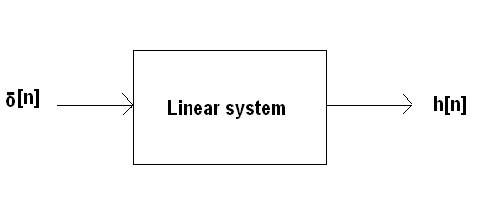EXPERIMENT NO.4

TITLE: TO WRITE A â€˜Câ€™ PROGRAM TO COMPUTE CONVOLUTION OF TWO SEQUENCES.

THEORY:

Convolution:-

Convolution is a mathematical way of combining two sequences or signals to form a third signal. It is the single most important technique in digital signal processing using the strategy of impulse deconvolution signals or systems are described by a signal called impulse response. Convolution is important because it relates the three signals and the impulse of interest, the input response, the output signal and the impulse response. This chapter presents convolution from two different algorithms called input side algorithm and output side algorithm. Convolution provides the mathematical frame work for DSP.

Lets summarize this way of understanding how a system changes an input signal into an output signal. First input signal can be received as a sealed and shifted delta function. Second the output resulting from each impulse is a sealed and shifted version of impulse response. Third the overall output signal can be found by adding these sealed and shifted impulse response.In other words if we know a system is impulse response then we can calculate what the output will be for any possible input signal. This means that we know everything about system. There is nothing more than that can be learned about the linear systems characteristics. (However, in later chapter we will show that this information can be represented in different forms). The impulse response goes by a different name in some applications. If the system being calculated considered is a filter, the impulse response is called as a filter. The convolution in image processing, the impulse response is called the point spread function.

While these terms are used in slightly different ways, they all mean the same thing. The signal produced by a system when input is delta function.Definition of delta function and impulse response of a delta function is a normalized impulse. All of its samples have a value of zero except for all the sample number zero, which have value one. The Greek letter delta *[n] is used to identify the delta function. The impulse response of a linear system, usually denoted by h[n] is the output of the system when the input is delta function.

Convolution is a formal mathematical representation just as multiplication, addition and integration. Addition takes two numbers and produces a 3 rd number. While convolution takes two signals and produces a third signal. Convolution is used in the mathematics of many systems, convolution is used in the mathematics of many systems, convolution takes place to describe the relationship between 3 signals of the notation interest, the input signal, the impulse response and the output signal. Fig shows the notations when convolution is used with linear system with an impulse response h[n] resulting in the output signal y[n].

In equation form: x[n] h[n] y[n]

Expressed in words, the input signal convoluted with response is equal to the output signal. Just as addition is represented by it. It is unfortunate that most programming languages use the star to indicate multiplication.

A star in computer program means multiplication, while a star in equation represents convolution

MATLAB CODE

Convolution of two sequences using c code

#include<stdio.h>

#include<conio.h>

#include<string.h>

#define N=10

void main( )

{

int mn,conlen,bitlen,I,j,l,temp,length,mul;

char gen,mes;

clrscr( );

printf(â€œ\t\t\t\t Convolution codes\nâ€);

printf(â€œ\n Enter the no of messege bitsâ€);

scanf(â€œ%dâ€,& mn);

printf(â€œ\n enter the constraint lengthâ€);

scanf(â€œ%dâ€,& conlen);

printf(â€œ\n Enter the no of outputs for each message bitsâ€);

scanf(â€œ%d â€œ,&bitlen);

for(i=0;i<bitlen;i++);

{

printf(â€œ\n Enter the %dth generating sequence in one row\nâ€,i+1);

scanf(â€œ%sâ€,&gen[i]);

}

printf(â€œ\n Enter the message sequenceâ€);

scanf(â€œ%s,&mes);

}

printf(â€œ\n Multiplexed output sequence isâ€);

i=0;

while(1)

{

for(j=0;j<bitlen;j++)

{

temp=length=0;

for(l=0;l<conlen;l++)

{
if(i-1)>=0&&(i-1)<mn)

{
length++;

if((gen[j][l]==â€™1â€™)&&(mes[i-1]==â€™1â€™)

mul=1;

else

mul=0;

temp=mul+temp;

}

}

if(temp%2==0)

printf(â€œ0â€);

else

printf(â€œ1â€);

}

printf(â€œ\tâ€);

if((j==bitlen)&&(length<=1)&&(i!=0));

break;

else

i++;

}

getch( );

}

Output

Convolution codes

Enter the no message bits 5

Enter the constraint length 3

Enter the no of outputs for each message bits 2

Enter the 1th generating sequence in one row

101

Enter the 2th generating sequence in one row

111

Enter the message sequence 1011

Multiplexed outpt sequence is 00 01 00 10 10 11 00

CONCLUSION: